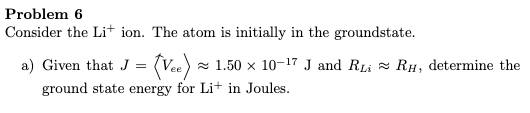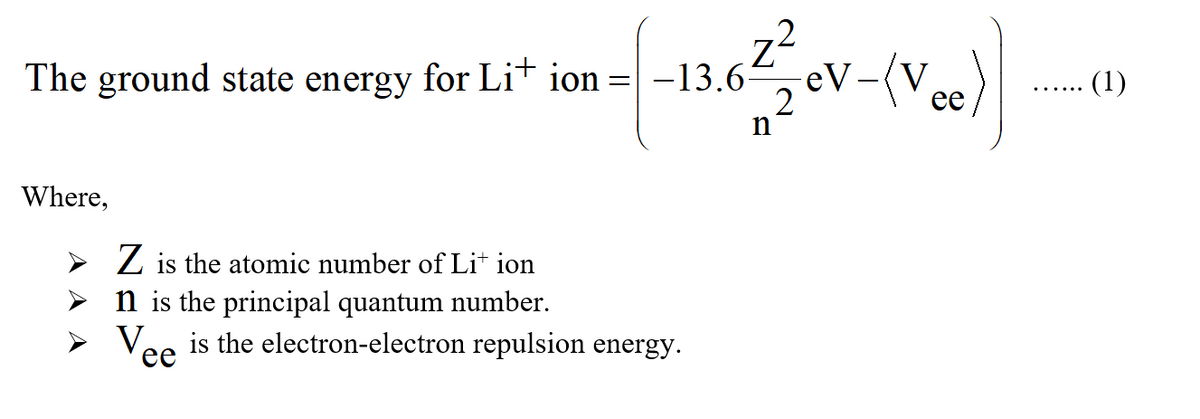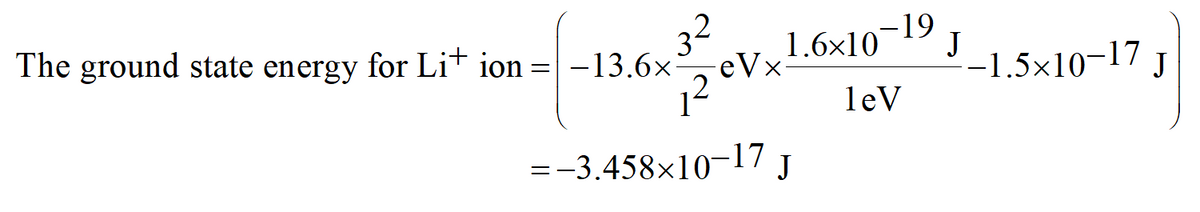# Problem 6Consider the Li+ ion. The atom is initially in the groundstate.a) Given that J = (Vee) x 1.50 × 10-17 J and RLi = RH, determine theground state energy for Li+ in Joules.

Question
2 viewshelp_outlineImage TranscriptioncloseProblem 6 Consider the Li+ ion. The atom is initially in the groundstate. a) Given that J = (Vee) x 1.50 × 10-17 J and RLi = RH, determine the ground state energy for Li+ in Joules. fullscreen
check_circle

Step 1

The species is said to be the hydrogen-like species if the one electron is present in the outer most shell. The lithium ion Li+ will be the hydrogen-like species because of the presence of one electron is present in the outer most shell.

Step 2

The expression to calculate the ground state energy for the Li+ ion is shown below:Step 3

The value of the atomic number Li+ ion is 3.

The value of the principal quantum number for the ground state is 1.

The value of the electron-electron repulsion energy is 1.5×10-17 J.

Substitute the value in equation (1)Therefore, the value of the ground state energy for the Li+ ion is -3....

### Want to see the full answer?

See Solution

#### Want to see this answer and more?

Solutions are written by subject experts who are available 24/7. Questions are typically answered within 1 hour.*

See Solution
*Response times may vary by subject and question.
Tagged in